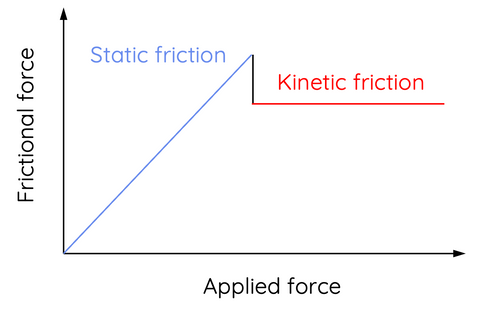# Forces: an Introduction

This topic is part of the HSC Physics course under the section Forces.

### HSC Physics Syllabus

• using Newton’s Laws of Motion, describe static and dynamic interactions between two or more objects and the changes that result from:
– a contact force
– a force mediated by fields

### What is a Forces? Types of Forces Explained

Forces are a fundamental concept in physics, responsible for the motion and interaction of objects.

### Understanding Forces

A force is a push or pull that acts upon an object. It can cause an object to accelerate, decelerate, or change direction. Forces are measured in units called newtons (N) and are represented by vectors, which have both magnitude and direction.

There are two main categories of forces: contact forces and field mediated forces. Contact forces occur when two objects are in direct physical contact, while field mediated forces act over a distance without any direct contact.

### Contact Forces

Contact forces arise due to the interaction between objects in direct contact. Some common examples of contact forces include:

Applied Force

Applied force refers to any force exerted by an object onto another object. For example, when you push a book across a table, you are applying a force to the book.

Normal Force

When an object is in contact with a surface, the surface exerts an upward force perpendicular to the surface, known as the normal force. This force prevents the object from penetrating the surface. The normal force acting on an object on a flat surface is equal in magnitude to the weight of the object.For example, when a box rests on a table, the table surface provides a normal force directly upwards on the box. The normal force vector is always perpendicular to the surface.

Friction

Friction is a force that opposes the relative motion or tendency of such motion between two surfaces in contact. Friction can be further classified into two types: static friction, which acts when the objects are stationary, and kinetic friction, which acts when the objects are in motion. Kinetic friction acts in the opposite direction to an object's velocity.

Friction is related to the normal force acting on the object, and is given by

$$f = \mu N$$

where f is the friction force, µ is the coefficient of friction and ﻿N﻿ is the normal force.Static friction always equals to the applied force until the applied force exceeds the maximum static friction that can be provided by the surface. When this occurs, the object begins to move and static friction is replaced by kinetic friction. The magnitude of kinetic friction is always lower than the maximum static friction provided by the surface.

Tension

Tension is the force exerted by a string, rope, or cable when it is stretched and subjected to an applied force. The tension force acts along the length of the material and is equal in magnitude at both ends.In the example above, two people apply forces on the rope in opposite directions. As a result of their applied forces, tension acts on them in the opposite direction, along the rope. The magnitude of tension acting on the two people is equal.

### Field Mediated Forces

Field mediated forces act over a distance without any direct contact between the objects involved. These forces are generated by fields that permeate space and can be classified into three main types:

Gravitational Force (Weight Force)

This force is the mutual attraction between any two objects with mass. It is responsible for the formation of planets, stars, and galaxies, as well as the motion of objects on Earth.

The mutual attraction between an object with mass and Earth is known as the weight force. The weight force on the surface of Earth is given by

$$F_{weight}=mg$$

where ﻿m ﻿is the object's mass, and ﻿g﻿ is the gravitational acceleration (or simply gravity). On the surface of Earth, ﻿g﻿ = 9.8 m s^{-2}.

Electromagnetic Force

This force is responsible for the interaction between charged particles. It can be attractive or repulsive, depending on the charges of the particles involved. Electromagnetic forces are responsible for many everyday phenomena, such as electricity, magnetism, and the behaviour of light. Electromagnetic force is discussed in Module 4: Electricity and Magnetism.

Nuclear Forces

These forces act within the nucleus of an atom, holding the protons and neutrons together. There are two types of nuclear forces: the strong nuclear force, which binds protons and neutrons together, and the weak nuclear force, which is responsible for certain types of radioactive decay. Nuclear forces are discussed in Module 8: From the Universe to The Atom.